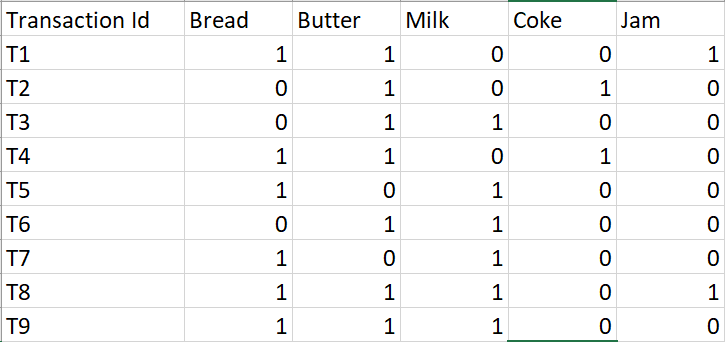GeeksforGeeks App
Open AppBrowser
Continue

# ML | ECLAT Algorithm

Prerequisites: Apriori Algorithm

The ECLAT algorithm stands for Equivalence Class Clustering and bottom-up Lattice Traversal. It is one of the popular methods of Association Rule mining. It is a more efficient and scalable version of the Apriori algorithm. While the Apriori algorithm works in a horizontal sense imitating the Breadth-First Search of a graph, the ECLAT algorithm works in a vertical manner just like the Depth-First Search of a graph. This vertical approach of the ECLAT algorithm makes it a faster algorithm than the Apriori algorithm.

How the algorithm work? :
The basic idea is to use Transaction Id Sets(tidsets) intersections to compute the support value of a candidate and avoiding the generation of subsets which do not exist in the prefix tree. In the first call of the function, all single items are used along with their tidsets. Then the function is called recursively and in each recursive call, each item-tidset pair is verified and combined with other item-tidset pairs. This process is continued until no candidate item-tidset pairs can be combined.

Let us now understand the above stated working with an example:-

Consider the following transactions record:-The above-given data is a boolean matrix where for each cell (i, j), the value denotes whether the j’th item is included in the i’th transaction or not. 1 means true while 0 means false.

We now call the function for the first time and arrange each item with it’s tidset in a tabular fashion:-

k = 1, minimum support = 2

ItemTidset
Bread{T1, T4, T5, T7, T8, T9}
Butter{T1, T2, T3, T4, T6, T8, T9}
Milk{T3, T5, T6, T7, T8, T9}
Coke{T2, T4}
Jam{T1, T8}

We now recursively call the function till no more item-tidset pairs can be combined:-

k = 2

ItemTidset
{Butter, Milk}{T3, T6, T8, T9}
{Butter, Coke}{T2, T4}
{Butter, Jam}{T1, T8}
{Milk, Jam}{T8}

k = 3

ItemTidset

k = 4

ItemTidset

We stop at k = 4 because there are no more item-tidset pairs to combine.

Since minimum support = 2, we conclude the following rules from the given dataset:-

Items BoughtRecommended Products